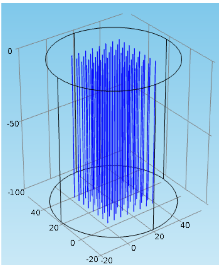# Presentazioni e Articoli Tecnici

## Numerically Generated g-functions for Ground Coupled Heat Pump Applications

J. Acuna, M. Fossa, P. Monzó
KTH Energy Technology, Stockholm, Sweden
Dime, University of Genova, Genova, Italy
Published in 2012

Ground-coupled heat pumps (GCHP) are successfully installed since at about 20 years in many countries to fulfill space conditioning requirements in building applications. In most cases the heat pump is connected to a system of vertical ground heat exchangers (as illustrated in Figure 1) where a fluid is circulated inside a system of pipes inserted in a deep borehole drilled in the soil. Drilling and installing Borehole Heat Exchangers (BHE) usually represents the highest cost in the thermal plant realization. Furthermore, the performance of the GCHP system are strictly related to the optimal design of the borefield, based on both short and long term dynamic analyses of the ground volume surrounding a system of BHEs. Medium to long term (decades) ground behavior analyses must take into consideration the time varying heat loads from/to the ground due to the building requirements, the borefield geometry, and the ground thermophysical properties. Probably, the most computational effective method for performing ground response yearly simulations even at very short time steps is the hybrid method of superposing in time the effects due to different heat loads by the way of pre-calculated temperature response factors or g-functions . A g-function represents the dimensionless temperature variation of a ground volume (with respect to the ground undisturbed and initial temperature and evaluated at the BHE periphery) to a stepwise, continuous and constant heat pulse lasting in time. Any particular borefield geometry has its own response and its own g-function. For very simple BHE geometries and under proper assumptions, some analytical g-function expressions are available (Ingersoll et al., 1954). When many BHEs are present, simple analytical solutions cannot be applied for long term analyses and dedicated g-functions have to be calculated. This calculation, which must include a time range up to decades, can be performed numerically, by solving the 3-D transient heat conduction differential equation. The first and most famous extensive research devoted to the calculation of g-functions is the one by , where the heat conduction equation is solved by imposing as a boundary condition, a constant heat temperature at every single BHE periphery. Another approach is again an hybrid method where a single BHE solution, the Finite Line Source Solution (FLS) is either superposed in time and in space, to describe the ground response of any BHE geometry. FLS generated g-functions are based on a different boundary condition (constant heat flux at BHE periphery) but in general they fit well the “reference”  functions. The purpose of this paper is to numerically calculate, for a few borefield geometries, g-function values by means of COMSOL simulations solving the 3-D problem with transient heat transfer equations and calculating the g-functions for the simulated geometries. The goal is to provide new information on reliability of FLS generated g-functions and to get a deep insight on the numerically generated ones, especially with reference to the “asymptotic” part of the g-function profiles and with reference to the boundary condition effects on temperature response factor shape.